Courses

# Lagrangian and Hamiltonian Formalism and Equations of Motion (Part - 1) - CSIR-NET Physical Sciences Physics Notes | EduRev

## Physics : Lagrangian and Hamiltonian Formalism and Equations of Motion (Part - 1) - CSIR-NET Physical Sciences Physics Notes | EduRev

The document Lagrangian and Hamiltonian Formalism and Equations of Motion (Part - 1) - CSIR-NET Physical Sciences Physics Notes | EduRev is a part of the Physics Course Physics for IIT JAM, UGC - NET, CSIR NET.
All you need of Physics at this link: Physics

Lagrange developed his approach in 1764 in a study of the libration of the moon, but it is best thought of as a general method of treating dynamics in terms of generalized coordinates for con guration space. It so transcends its origin that the Lagrangian is considered the fundamental ob ject which describes a quantum fi eld theory.

Hamilton’s approach arose in 1835 in his uni cation of the language of optics and mechanics. It too had a usefulness far beyond its origin, and the Hamiltonian is now most familiar as the operator in quantum mechanics which determines the evolution in time of the wave function.

We begin by deriving Lagrange’s equation as a simple change of coordinates in an unconstrained system, one which is evolving according to Newton’s laws with force laws given by some potential. Lagrangian mechanics is also and especially useful in the presence of constraints, so we will then extend the formalism to this more general situation.

Lagrangian for unconstrained systems

For a collection of particles with conservative forces described by a potential, we have in inertial cartesian coordinates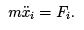The left hand side of this equation is determined by the kinetic energy function as the time derivative of the momentum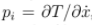while the right hand side is a derivative of the potential energy,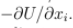As T is independent of xi and U is independent of  ̇xi in these coordinates, we can write both sides in terms of the Lagrangian L = T − U, which is then a function of both the coordinates and their velocities. Thus we have established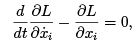which, once we generalize it to arbitrary coordinates, will be known as Lagrange’s equation. Note that we are treating L as a function of the 2N

independent variables xi and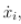so that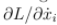means vary one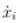holding all the other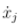and all the xk fixed. Making this particular combination of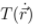with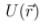to get the more complicated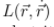seems an artificial  construction for the inertial cartesian coordinates, but it has the advantage of preserving the form of Lagrange’s equations for any set of generalized coordinates.

As we did in section 1.3.3, we assume we have a set of generalized coordinates {q} which parameterize all of coordinate space, so that each point may be described by the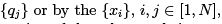and thus each set may be thought of as a function of the other, and time: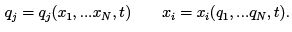(2.1)

We may consider L as a function1 of the generalized coordinates qj and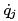and ask whether the same expression in these coordinates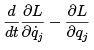also vanishes. The chain rule tells us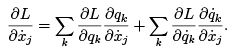(2.2)

The first term vanishes because qdepends only on the coordinates xk and t, but not on the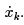From the inverse relation to (1.10),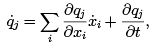(2.3)

we have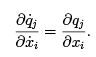Using this in (2.2),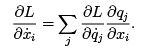(2.4)

Lagrange’s equation involves the time derivative of this. Here what is meant is not a partial derivative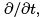holding the point in con guration space xed, but rather the derivative along the path which the system takes as it moves through con guration space. It is called the stream derivative,a name which comes from ﬂuid mechanics, where it gives the rate at which some property de ned throughout the ﬂuid,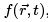changes for a xed element of ﬂuid as the ﬂuid as a whole ﬂows. We write it as a total derivative to indicate that we are following the motion rather than evaluating the rate of change at a xed point in space, as the partial derivative does.

For any function f (x; t) of extended con guration space, this total time derivative is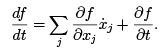(2.5)

Using Leibnitz’ rule on (2.4) and using (2.5) in the second term, we nd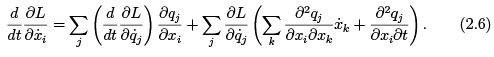On the other hand, the chain rule also tells us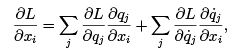where the last term does not necessarily vanish, asin general depends on both the coordinates and velocities. In fact, from 2.3,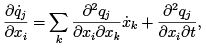so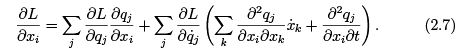Lagrange’s equation in cartesian coordinates says (2.6) and (2.7) are equal, and in subtracting them the second terms cancel2,so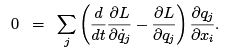The matrix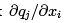is nonsingular, as it has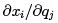as its inverse, so we have derived Lagrange’s Equation in generalized coordinates: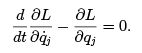Thus we see that Lagrange’s equations are form invariant under changes of the generalized coordinates used to describe the con guration of the system.
It is primarily for this reason that this particular and peculiar combination of kinetic and potential energy is useful. Note that we implicity assume the Lagrangian itself transformed like a scalar, in that its value at a given physical point of con guration space is independent of the choice of generalized coordinates that describe the point. The change of coordinates itself (2.1) is called a point transformation.

Lagrangian for Constrained Systems

We now wish to generalize our discussion to include contraints. At the same time we will also consider possibly nonconservative forces.  we often have a system with internal forces whose effect is better understood than the forces themselves, with which we may not be concerned. We will assume the constraints are holonomic, expressible as k real functions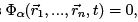which are somehow enforced by constraint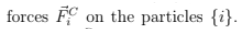There may also be other forces, which we will call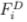and will treat as having a dynamical effect. These are given by known functions of the configuration and time, possibly but not necessarily in terms of a potential.

This distinction will seem arti cial without examples, so it would be well to keep these two in mind. In each of these cases the full con guration space is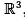, but the constraints restrict the motion to an allowed subspace of extended con guration space.

1. we discussed a mass on a light rigid rod, the other end of which is xed at the origin. Thus the mass is constrained to have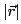= L, and the allowed subspace of con guration space is the surface of a sphere, independent of time. The rod exerts the constraint force to avoid compression or expansion. The natural assumption to make is that the force is in the radial direction, and therefore has no component in the direction of allowed motions, the tangential directions. That is, for all allowed displacements,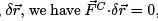and the constraint force does no work.

2. Consider a bead free to slide without friction on the spoke of a rotating bicycle wheel, rotating about a xed axis at xed angular velocity ω.
That is, for the polar angle θ of inertial coordinates,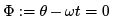is a constraint, but the r coordinate is unconstrained. Here the allowed subspace is not time independent, but is a helical sort of structure in extended con guration space. We expect the force exerted by the spoke on the bead to be in the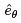direction. This is again perpendicular to any virtual displacement, by which we mean an allowed change in con guration at a xed time. It is important to distinguish this virtual displacement from a small segment of the tra jectory of the particle. In this case a virtual displacement is a change in r without a change in θ, and is perpendicular to. So again, we have the \net virtual work" of the constraint forces is zero. It is important to note that this does not mean that the net real work is zero. In a small time interval, the displacement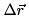includes a component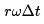in the tangential direction, and the force of constraint does do work!

We will assume that the constraint forces in general satisfy this restriction that no net virtual work is done by the forces of constraint for any possible virtual displacement. Newton’s law tells us that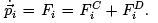We can multiply by an arbitrary virtual displacement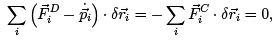where the rst equality would be true even if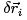did not satisfy the constraints, but the second requiresto be an allowed virtual displacement.
Thus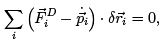(2.8)

which is known as D’Alembert’s Principle. This gives an equation which determines the motion on the constrained subspace and does not involve the unspeci ed forces of constraint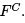We drop the superscript D from now on.
Suppose we know generalized coordinates q1,..........,qN which parameterize the constrained subspace, which means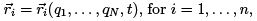are known functions and the Nq’s are independent. There are N =3n − k of these independent coordinates, where k is the number of holonomic constraints. Then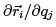is no longer an invertable, or even square, matrix, but we still have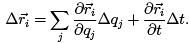For the velocity of the particle, divide this by Δt, giving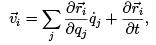(2.9)

but for a virtual displacement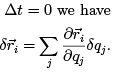Differentiating (2.9) we note that,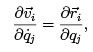(2.10)

and also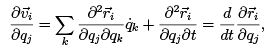(2.11)

where the last equality comes from applying (2.5), with coordinates qj rather than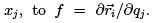The first term in the equation (2.8) stating D’Alembert’s principle is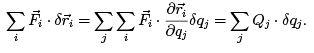The generalized force Qj has the same form as in the unconstrained case, as given by (1.9), but there are only as many of them as there are unconstrained degrees of freedom.
The second term of (2.8) involves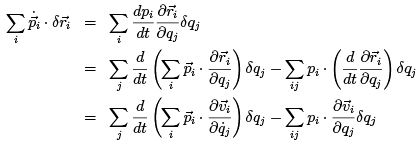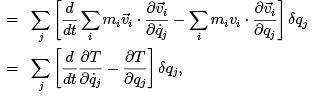where we used (2.10) and (2.11) to get the third line. Plugging in the expressions we have found for the two terms in D’Alembert’s Principle,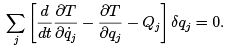We assumed we had a holonomic system and the q’s were all independent, so this equation holds for arbitrary virtual displacements δqj , and therefore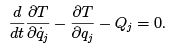(2.12)

Now let us restrict ourselves to forces given by a potential, with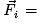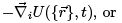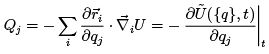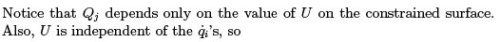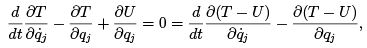or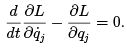(2.13)

This is Lagrange’s equation, which we have now derived in the more general context of constrained systems.

2.2.1 Some examples of the use of Lagrangians

Atwood’s machine

Atwood’s machine consists of two blocks of mass m1 and m2 attached by an inextensible cord which suspends them from a pulley of moment of inertia I with frictionless bearings. The kinetic energy is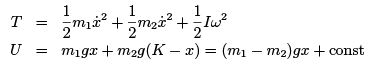where we have used the xed length of the cord to conclude that the sum of the heights of the masses is a constant K . We assume the cord does not slip on the pulley, so the angular velocity of the pulley is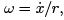and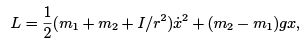and Lagrange’s equation gives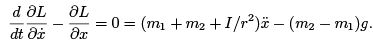Notice that we set up our system in terms of only one degree of freedom, the height of the rst mass. This one degree of freedom parameterizes the line which is the allowed subspace of the unconstrained con guration space, a three dimensional space which also has directions corresponding to the angle of the pulley and the height of the second mass. The constraints restrict these three variables because the string has a xed length and does not slip on the pulley. Note that this formalism has permitted us to solve the problem without solving for the forces of constraint, which in this case are the tensions in the cord on either side of the pulley.

As a second example, reconsider the bead on the spoke of a rotating bicycle wheel. In section (1.3.4) we saw that the kinetic energy is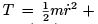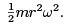If there are no forces other than the constraint forces,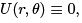and the Lagrangian is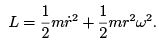The equation of motion for the one degree of freedom is easy enough: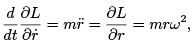which looks like a harmonic oscillator with a negative spring constant, so the solution is a real exponential instead of oscillating,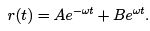The velocity-independent term in T acts just like a potential would, and can in fact be considered the potential for the centrifugal force. But we see that the total energy T is not conserved but blows up as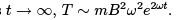This is because the force of constraint, while it does no virtual work, does do real work.

Mass on end of gimballed rod

Finally, let us consider the mass on the end of the gimballed rod. The allowed subspace is the surface of a sphere, which can be parameterized by an azimuthal angle φ and the polar angle with the upwards direction, θ,in terms of which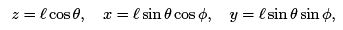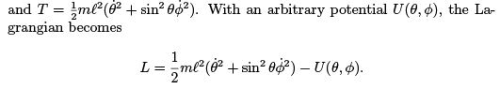From the two independent variables θ; φ there are two Lagrange equations of motion,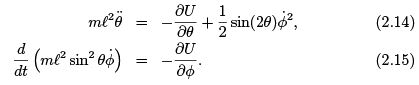Notice that this is a dynamical system with two coordinates, similar to ordinary mechanics in two dimensions, except that the mass matrix, while diagonal, is coordinate dependent, and the space on which motion occurs is not an in nite ﬂat plane, but a curved two dimensional surface, that of a sphere.
These two distinctions are connected|the coordinates enter the mass matrix because it is impossible to describe a curved space with unconstrained cartesian coordinates.

Often the potential U(θ, φ) will not actually depend on φ, in which case Eq. 2.15 tells us ml2 sin2 θφ ̇ is constant in time.

Offer running on EduRev: Apply code STAYHOME200 to get INR 200 off on our premium plan EduRev Infinity!

159 docs

,

,

,

,

,

,

,

,

,

,

,

,

,

,

,

,

,

,

,

,

,

;